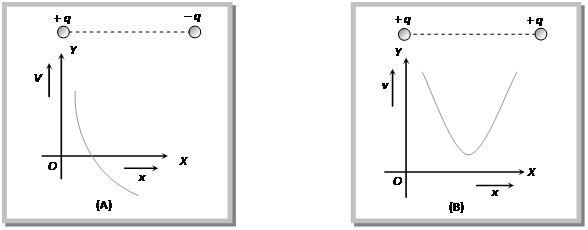# CBSE Physics for Class 12 | Electrostatics | Electric Potential

## Electric Potential

### (1) Definition of Electric Potential.:

Potential at a point in a field is defined as the amount of work done in bringing a unit positive test charge, from infinity to that point along any arbitrary path (infinity is point of zero potential). Electric potential is a scalar quantity, it is denoted by V;$\displaystyle V=\frac{W}{{{q}_{\mathbf{0}}}}$

### (2) Unit and dimensional formula of Electric Potential.:

S. I. unit –$\displaystyle \frac{Joule}{Coulomb}=volt$ C.G.S. unit – Stat volt (e.s.u.); 1 volt$\displaystyle =\frac{\mathbf{1}}{\mathbf{300}}$ Stat volt Dimension of electric potential are$\displaystyle [V]=[M{{L}^{2}}{{T}^{-3}}{{A}^{-1}}]$

### (3) Types of electric potential :

According to the nature of charge potential is of two types

(i) Positive potential : Due to positive charge.

(ii) Negative potential : Due to negative charge.

### (4) Potential of a system of point charges :

Consider P is a point at which net electric potential is to be determined due to several charges. So net potential at P$\displaystyle V=k\frac{{{Q}_{1}}}{{{r}_{1}}}+k\frac{{{Q}_{2}}}{{{r}_{2}}}+k\frac{{{Q}_{3}}}{{{r}_{3}}}+k\frac{\left( -{{Q}_{4}} \right)}{{{r}_{4}}}+...$

In general$\displaystyle V=\sum\limits_{i=1}^{X}{\frac{k{{Q}_{i}}}{{{r}_{i}}}}$

Note :

• At the centre of two equal and opposite charge V = 0
but$\displaystyle E\ne 0$
• At the centre of the line joining two equal and similar charge$\displaystyle V\ne 0,\,E=0$

### (5) Electric potential due to a continuous charge distribution :

The potential due to a continuous charge distribution is the sum of potentials of all the infinitesimal charge elements in which the distribution may be divided i.e.,$\displaystyle V=\int{dV},\,\,\,\,=\int{\frac{dQ}{4\pi {{\varepsilon }_{0}}r}}$

### (6) Graphical representation of electric potential :

When we move from a positive charge towards an equal negative charge along the line joining the two then initially potential decreases in magnitude and at centre become zero, but this potential is throughout positive because when we are nearer to positive charge, overall potential must be positive.

When we move from centre towards the negative charge then though potential remain always negative but increases in magnitude fig. (A).

As one move from one charge to other when both charges are like, the potential first decreases, at centre become minimum and then increases Fig. (B).### (7) Potential difference :

In an electric field potential difference between two points A and B is defined as equal to the amount of work done (by external agent) in moving a unit positive charge from point A to point B.

i.e.,$\displaystyle {{V}_{B}}-{{V}_{A}}=\frac{W}{{{q}_{0}}}$ in general$\displaystyle W=Q.\,\Delta V$;$\displaystyle \Delta V=$Potential difference through which charge Q moves.

This site uses Akismet to reduce spam. Learn how your comment data is processed.